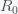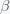# How Much Covid-19 Testing Do We Need?

Posted on the 29 May 2020 by Ccc1685 @ccc1685

There is a simple way to estimate how much SARS-CoV-2 PCR testing we need to start diminishing the COVID-19 pandemic. Suppose we test everyone at a rate, with a PCR test with 100% sensitivity, which means we do not miss anyone who is positive but we could have false positives. The number of positives we will find is, whereis the prevalence of infectious individuals in a given population. If positive individuals are isolated from the rest of the population until they are no longer infectious with probability, then the rate of reduction in prevalence is. To reduce the pandemic, this number needs to be higher than the rate of pandemic growth, which is given by, whereis the fraction of the population susceptible to SARS-CoV-2 infection andis the rate of transmission from an infected individual to a susceptible upon contact. Thus, to reduce the pandemic, we need to test at a rate higher than.

In the initial stages of the pandemicis one and, whereis the mean reproduction number, which is probably around 3.7 andis the mean rate of becoming noninfectious, which is probably around 10 to 20 days. This gives an estimate ofto be somewhere around 0.3 per day. Thus, in the early stages of the pandemic, we would need to test everyone at least two or three times per week, provided positives are isolated. However, if people wear masks and avoid crowds thencould be reduced. If we can get it smaller then we can test less frequently. Currently, the global average ofis around one, so that would mean we need to test every two or three weeks. If positives don't isolate with high probability, we need to test at a higher rate to compensate. This threshold rate will also go down asgoes down.

In fact, you can just test randomly at rateand monitor the positive rate. If the positive rate trends downward then you are testing enough. If it is going up then test more. In any case, we may need less testing capability than we originally thought, but we do need to test the entire population and not just suspected cases.

See more